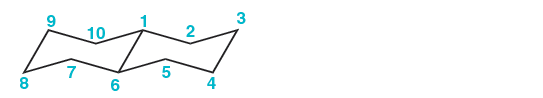# Problem: Below is the numbered skeleton of trans-decalin: Identify whether each of the following substituents would be in an equatorial position or an axial position: (a) A group at the C-2 position, pointing UP (b) A group at the C-3 position, pointing DOWN (c) A group at the C-4 position, pointing DOWN (d) A group at the C-7 position, pointing DOWN (e) A group at the C-8 position, pointing UP (f ) A group at the C-9 position, pointing UP

🤓 Based on our data, we think this question is relevant for Professor Salmon's class at UMN.

###### Problem Details

Below is the numbered skeleton of trans-decalin:

Identify whether each of the following substituents would be in an equatorial position or an axial position:

(a) A group at the C-2 position, pointing UP

(b) A group at the C-3 position, pointing DOWN

(c) A group at the C-4 position, pointing DOWN

(d) A group at the C-7 position, pointing DOWN

(e) A group at the C-8 position, pointing UP

(f ) A group at the C-9 position, pointing UPWhat scientific concept do you need to know in order to solve this problem?

Our tutors have indicated that to solve this problem you will need to apply the Decalin concept. You can view video lessons to learn Decalin. Or if you need more Decalin practice, you can also practice Decalin practice problems.

What is the difficulty of this problem?

Our tutors rated the difficulty ofBelow is the numbered skeleton of trans-decalin: Identify w...as high difficulty.

How long does this problem take to solve?

Our expert Organic tutor, Chris took 2 minutes and 51 seconds to solve this problem. You can follow their steps in the video explanation above.

What professor is this problem relevant for?

Based on our data, we think this problem is relevant for Professor Salmon's class at UMN.

What textbook is this problem found in?

Our data indicates that this problem or a close variation was asked in Organic Chemistry - Klein 1st Edition. You can also practice Organic Chemistry - Klein 1st Edition practice problems.